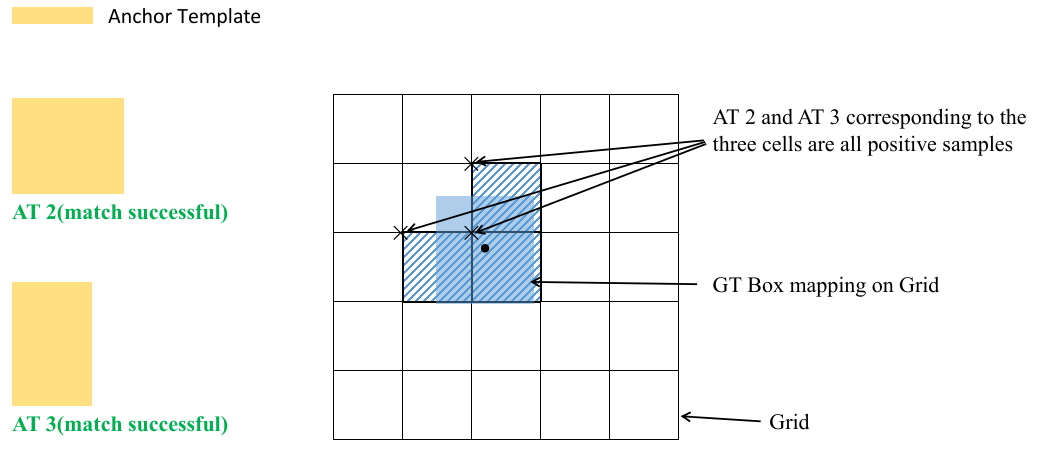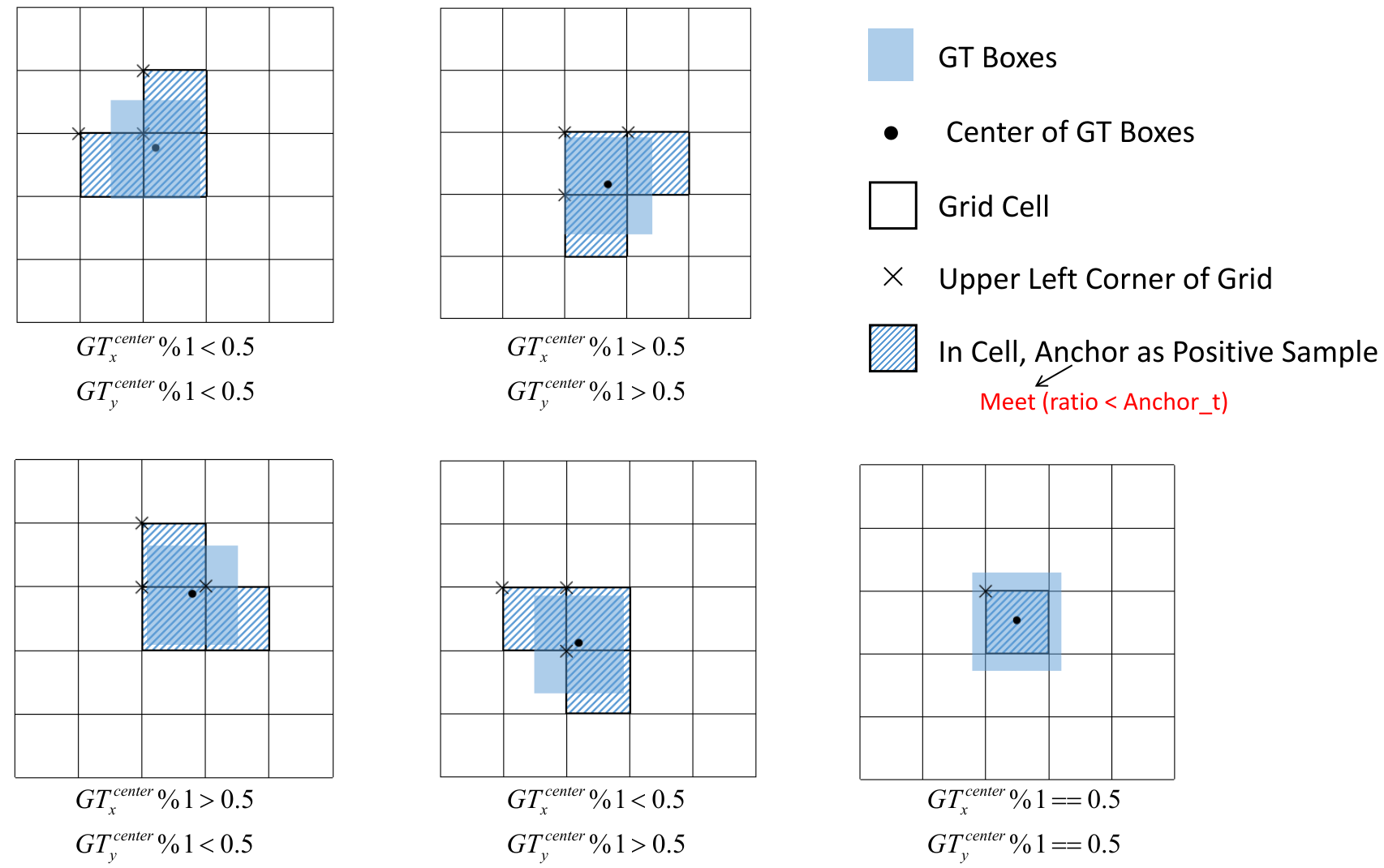## 0 前言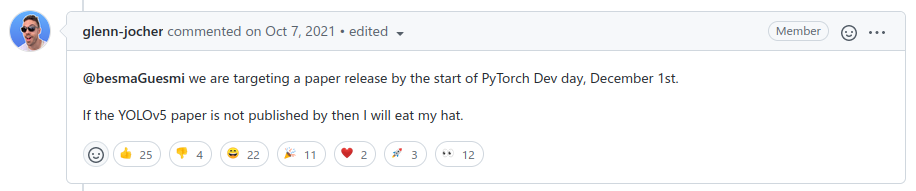(⊙o⊙)…，emmm，但这都2022年了，也不知道他的帽子是啥味儿。过了他承诺的发表期限后，很多人还去该issue下表示"关怀"，问啥时候吃帽子，下面这位大哥给我整笑了。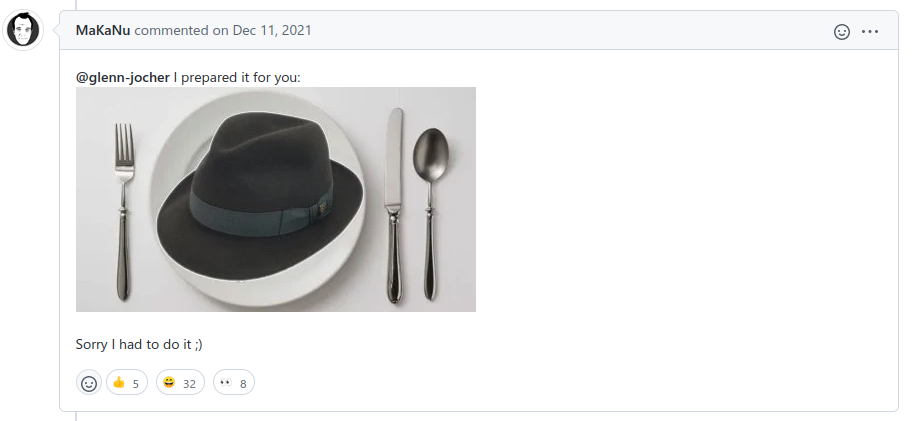Modelsize
(pixels)
mAPval
0.5:0.95
mAPval
0.5
Speed
CPU b1
(ms)
Speed
V100 b1
(ms)
Speed
V100 b32
(ms)
params
(M)
FLOPs
@640 (B)
YOLOv5n64028.045.7456.30.61.94.5
YOLOv5s64037.456.8986.40.97.216.5
YOLOv5m64045.464.12248.21.721.249.0
YOLOv5l64049.067.343010.12.746.5109.1
YOLOv5x64050.768.976612.14.886.7205.7
YOLOv5n6128036.054.41538.12.13.24.6
YOLOv5s6128044.863.73858.23.612.616.8
YOLOv5m6128051.369.388711.16.835.750.0
YOLOv5l6128053.771.3178415.810.576.8111.4
YOLOv5x6
+ TTA
1280
1536
55.0
55.8
72.7
72.7
3136
-
26.2
-
19.4
-
140.7
-
209.8
-

## 1 网络结构

• Backbone: New CSP-Darknet53
• Neck: SPPF, New CSP-PAN
• Head: YOLOv3 Head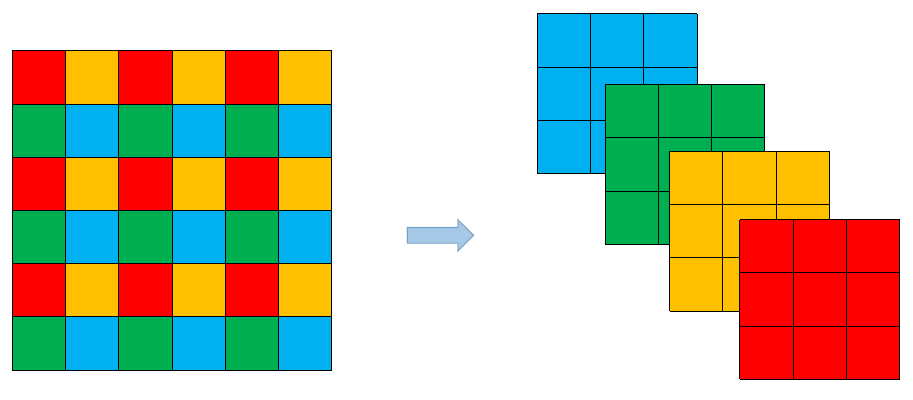Neck部分的变化还是相对较大的，首先是将SPP换成成了SPPFGlenn Jocher自己设计的），这个改动我个人觉得还是很有意思的，两者的作用是一样的，但后者效率更高。SPP结构如下图所示，是将输入并行通过多个不同大小的MaxPool，然后做进一步融合，能在一定程度上解决目标多尺度问题。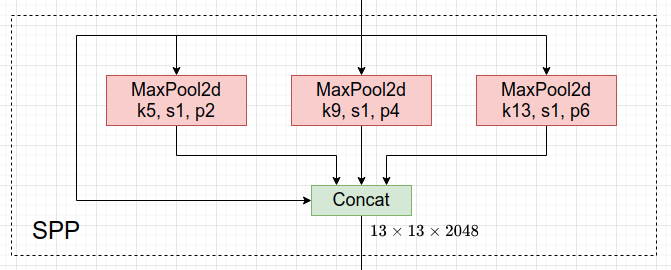SPPF结构是将输入串行通过多个5x5大小的MaxPool层，这里需要注意的是串行两个5x5大小的MaxPool层是和一个9x9大小的MaxPool层计算结果是一样的，串行三个5x5大小的MaxPool层是和一个13x13大小的MaxPool层计算结果是一样的。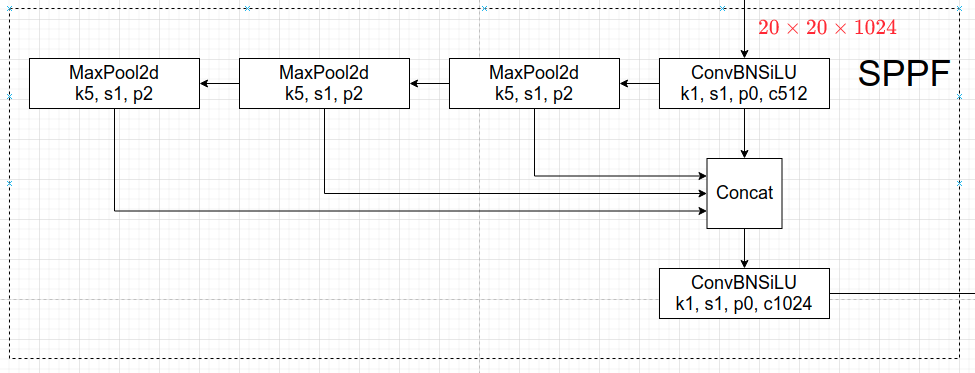import time
import torch
import torch.nn as nn

class SPP(nn.Module):
def __init__(self):
super().__init__()
self.maxpool1 = nn.MaxPool2d(5, 1, padding=2)
self.maxpool2 = nn.MaxPool2d(9, 1, padding=4)
self.maxpool3 = nn.MaxPool2d(13, 1, padding=6)

def forward(self, x):
o1 = self.maxpool1(x)
o2 = self.maxpool2(x)
o3 = self.maxpool3(x)

class SPPF(nn.Module):
def __init__(self):
super().__init__()
self.maxpool = nn.MaxPool2d(5, 1, padding=2)

def forward(self, x):
o1 = self.maxpool(x)
o2 = self.maxpool(o1)
o3 = self.maxpool(o2)

def main():
input_tensor = torch.rand(8, 32, 16, 16)
spp = SPP()
sppf = SPPF()
output1 = spp(input_tensor)
output2 = sppf(input_tensor)

print(torch.equal(output1, output2))

t_start = time.time()
for _ in range(100):
spp(input_tensor)
print(f"spp time: {time.time() - t_start}")

t_start = time.time()
for _ in range(100):
sppf(input_tensor)
print(f"sppf time: {time.time() - t_start}")

if __name__ == '__main__':
main()


True
spp time: 0.5373051166534424
sppf time: 0.20780706405639648


Neck部分另外一个不同点就是New CSP-PAN了，在YOLOv4中，NeckPAN结构是没有引入CSP结构的，但在YOLOv5中作者在PAN结构中加入了CSP。详情见上面的网络结构图，每个C3模块里都含有CSP结构。在Head部分，YOLOv3, v4, v5都是一样的，这里就不讲了。

## 2 数据增强

• Mosaic，将四张图片拼成一张图片，讲过很多次了• Copy paste，将部分目标随机的粘贴到图片中，前提是数据要有segments数据才行，即每个目标的实例分割信息。下面是Copy paste原论文中的示意图。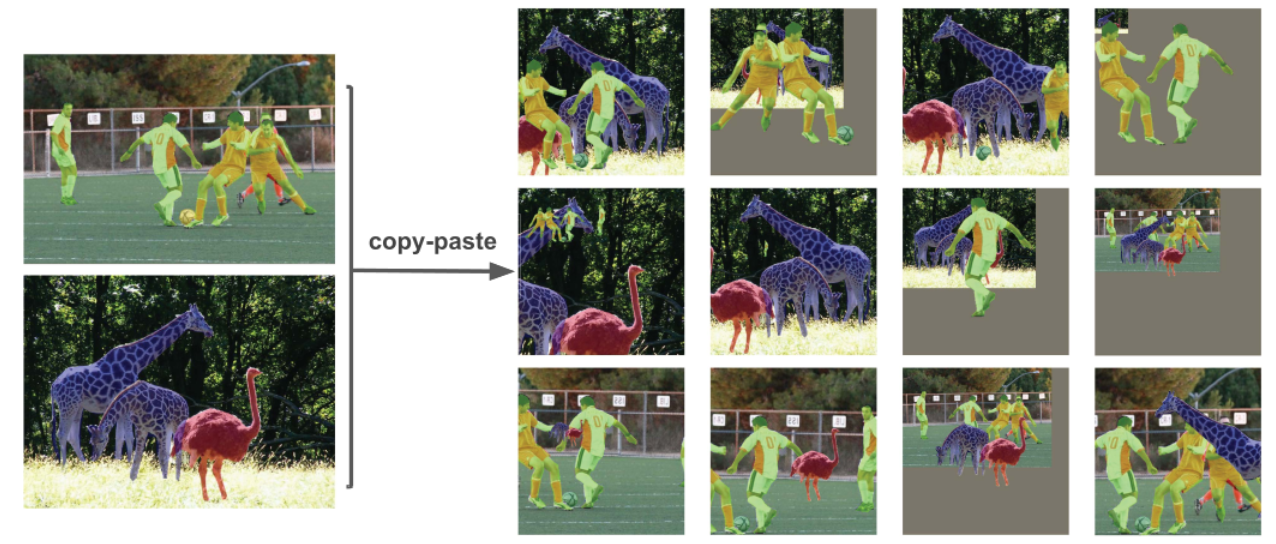• Random affine(Rotation, Scale, Translation and Shear)，随机进行仿射变换，但根据配置文件里的超参数发现只使用了ScaleTranslation即缩放和平移。• MixUp，就是将两张图片按照一定的透明度融合在一起，具体有没有用不太清楚，毕竟没有论文，也没有消融实验。代码中只有较大的模型才使用到了MixUp，而且每次只有10%的概率会使用到。• Albumentations，主要是做些滤波、直方图均衡化以及改变图片质量等等，我看代码里写的只有安装了albumentations包才会启用，但在项目的requirements.txt文件中albumentations包是被注释掉了的，所以默认不启用。

• Augment HSV(Hue, Saturation, Value)，随机调整色度，饱和度以及明度。• Random horizontal flip，随机水平翻转## 3 训练策略

• Multi-scale training(0.5~1.5x)，多尺度训练，假设设置输入图片的大小为 640 × 640 640 \times 640 ，训练时采用尺寸是在 0.5 × 640 ∼ 1.5 × 640 0.5 \times 640 \sim 1.5 \times 640 之间随机取值，注意取值时取得都是32的整数倍（因为网络会最大下采样32倍）。
• AutoAnchor(For training custom data)，训练自己数据集时可以根据自己数据集里的目标进行重新聚类生成Anchors模板。
• Warmup and Cosine LR scheduler，训练前先进行Warmup热身，然后在采用Cosine学习率下降策略。
• EMA(Exponential Moving Average)，可以理解为给训练的参数加了一个动量，让它更新过程更加平滑。
• Mixed precision，混合精度训练，能够减少显存的占用并且加快训练速度，前提是GPU硬件支持。
• Evolve hyper-parameters，超参数优化，没有炼丹经验的人勿碰，保持默认就好。

## 4 其他

### 4.1 损失计算

YOLOv5的损失主要由三个部分组成：

• Classes loss，分类损失，采用的是BCE loss，注意只计算正样本的分类损失。
• Objectness lossobj损失，采用的依然是BCE loss，注意这里的obj指的是网络预测的目标边界框与GT Box的CIoU。这里计算的是所有样本的obj损失。
• Location loss，定位损失，采用的是CIoU loss，注意只计算正样本的定位损失。

L o s s = λ 1 L c l s + λ 2 L o b j + λ 3 L l o c Loss=\lambda_1 L_{cls} + \lambda_2 L_{obj} + \lambda_3 L_{loc}

### 4.2 平衡不同尺度的损失

L o b j = 4.0 ⋅ L o b j s m a l l + 1.0 ⋅ L o b j m e d i u m + 0.4 ⋅ L o b j l a r g e L_{obj} = 4.0 \cdot L_{obj}^{small} + 1.0 \cdot L_{obj}^{medium} + 0.4 \cdot L_{obj}^{large}

### 4.3 消除Grid敏感度• t x t_x 是网络预测的目标中心 x x 坐标偏移量（相对于网格的左上角）
• t y t_y 是网络预测的目标中心 y y 坐标偏移量（相对于网格的左上角）
• c x c_x 是对应网格左上角的 x x 坐标
• c y c_y 是对应网格左上角的 y y 坐标
• σ \sigma Sigmoid激活函数，将预测的偏移量限制在0到1之间，即预测的中心点不会超出对应的Grid Cell区域

b x = ( 2 ⋅ σ ( t x ) − 0.5 ) + c x b y = ( 2 ⋅ σ ( t y ) − 0.5 ) + c y b_x = (2 \cdot \sigma(t_x) - 0.5) + c_x \\ b_y = (2 \cdot \sigma(t_y) - 0.5) + c_y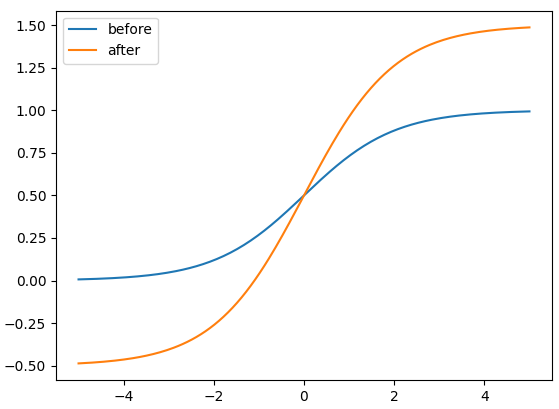b w = p w ⋅ e t w b h = p h ⋅ e t h b_w = p_w \cdot e^{t_w} \\ b_h = p_h \cdot e^{t_h}

b w = p w ⋅ ( 2 ⋅ σ ( t w ) ) 2 b h = p h ⋅ ( 2 ⋅ σ ( t h ) ) 2 b_w = p_w \cdot (2 \cdot \sigma(t_w))^2 \\ b_h = p_h \cdot (2 \cdot \sigma(t_h))^2

The original yolo/darknet box equations have a serious flaw. Width and Height are completely unbounded as they are simply out=exp(in), which is dangerous, as it can lead to runaway gradients, instabilities, NaN losses and ultimately a complete loss of training.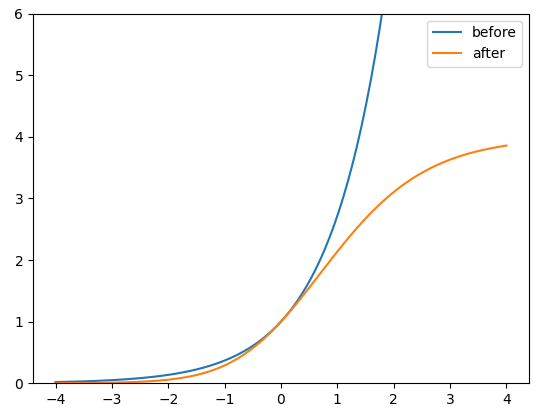### 4.4 匹配正样本(Build Targets)

r w = w g t / w a t r h = h g t / h a t r_w = w_{gt} / w_{at} \\ r_h = h_{gt} / h_{at} \\

r w m a x = m a x ( r w , 1 / r w ) r h m a x = m a x ( r h , 1 / r h ) r_w^{max} = max(r_w, 1 / r_w) \\ r_h^{max} = max(r_h, 1 / r_h)

r m a x = m a x ( r w m a x , r h m a x ) r^{max} = max(r_w^{max}, r_h^{max})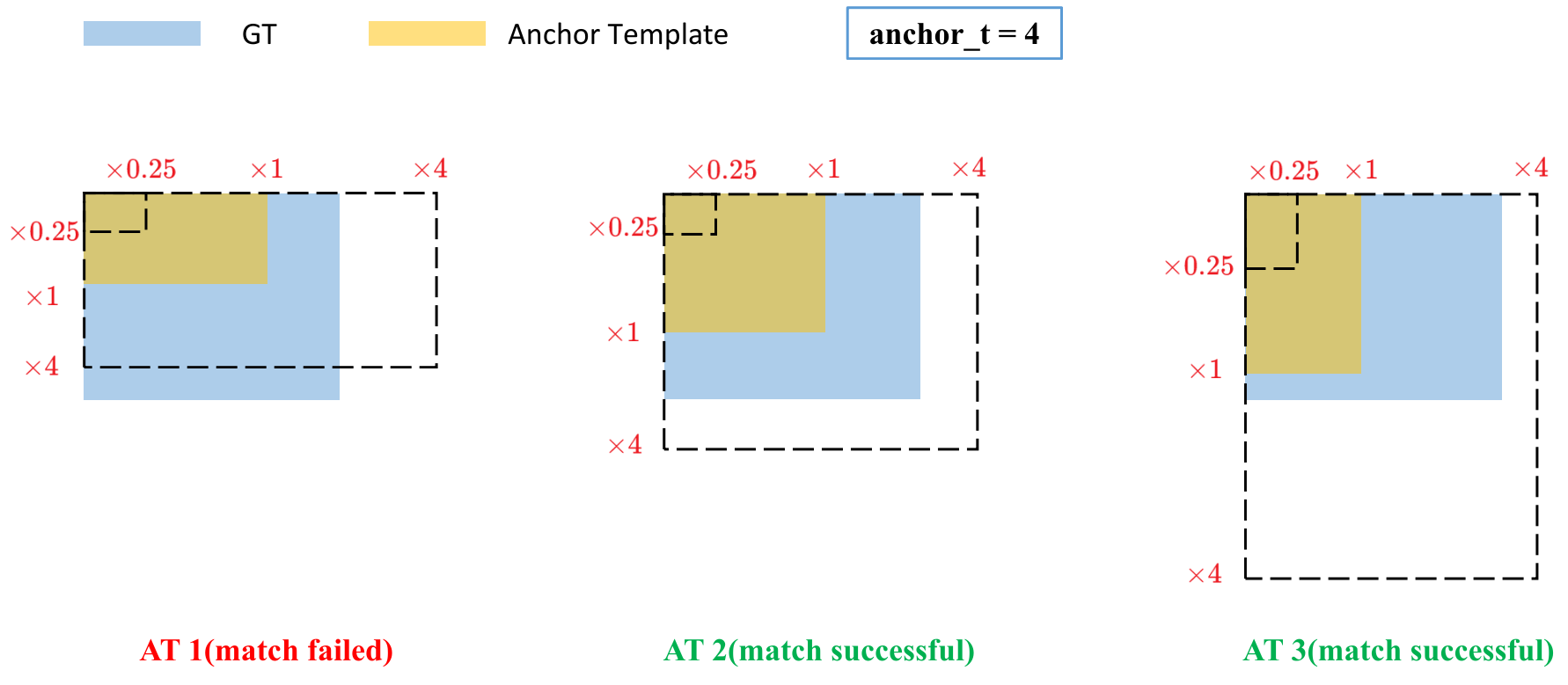• GT投影到对应预测特征层上，根据GT的中心点定位到对应Cell，注意图中有三个对应的Cell。因为网络预测中心点的偏移范围已经调整到了 ( − 0.5 , 1.5 ) (-0.5, 1.5) ，所以按理说只要Grid Cell左上角点距离GT中心点在 ( − 0.5 , 1.5 ) (−0.5,1.5) 范围内它们对应的Anchor都能回归到GT的位置处。这样会让正样本的数量得到大量的扩充。
• 则这三个Cell对应的AT2AT3都为正样本。